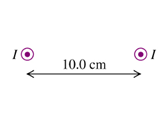# Problem: Two long, straight, parallel wires, 10.0 cm apart carry equal 4.00-A currents in the same direction, as shown in the figure.Part AFind the magnitude of the magnetic field at point P1, midway between the wires.Part BWhat is the direction?a. to the leftb. to the rightc. upwardd. downwarde. no field

###### FREE Expert Solution

Magnetic field around a conductor:

$\overline{){\mathbf{B}}{\mathbf{=}}\frac{{\mathbf{\mu }}_{\mathbf{0}}\mathbf{i}}{\mathbf{2}\mathbf{\pi }\mathbf{r}}}$

Where i is the current through the conductor and r is the distance from the conductor.

79% (163 ratings)###### Problem Details

Two long, straight, parallel wires, 10.0 cm apart carry equal 4.00-A currents in the same direction, as shown in the figure.Part A

Find the magnitude of the magnetic field at point P1, midway between the wires.

Part B

What is the direction?

a. to the left

b. to the right

c. upward

d. downward

e. no field